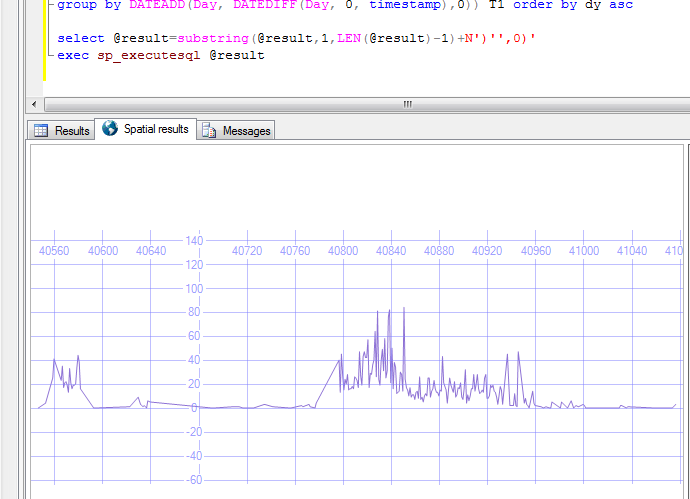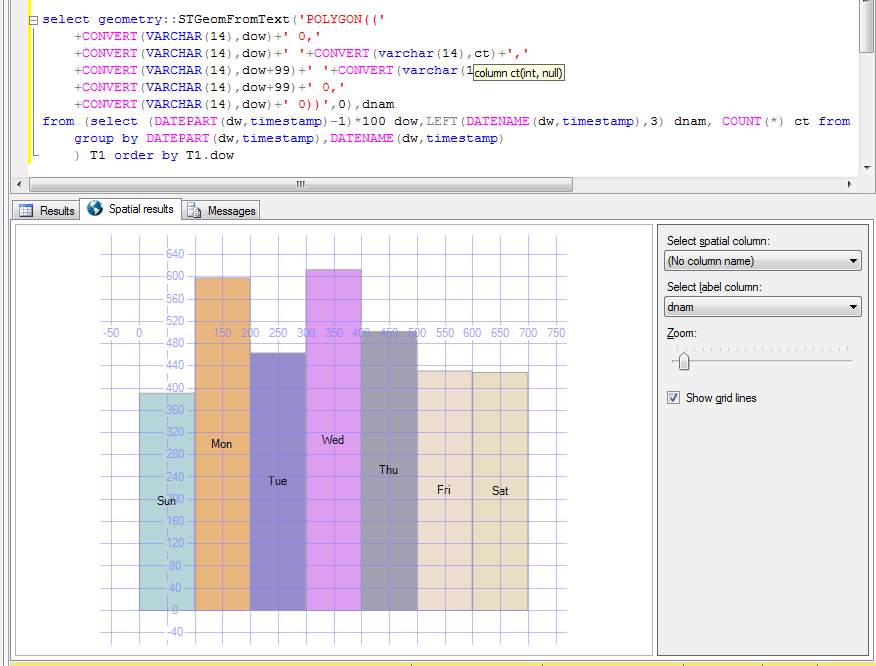# Lateral Thinking with SQL Spatial Visualisation

It just occurred to me that SQL’s geometry type can be used like a canvas to visualise nonspatial data in SQL Server – to make graphs etc.

Sure there are other tools, more appropriate tools (like Excel) but you don’t always have them to hand or set up. For instance all I wanted was a quick visualisation of the number of calls one of our customers was taking over time.

```declare @result nvarchar(max)=N'select geometry::STGeomFromText(''LINESTRING('

select @result=@result+convert(nvarchar(14),convert(float,dy))+' '+CONVERT(nvarchar(14),ct)+', '
from
(select DATEADD(Day, DATEDIFF(Day, 0, timestamp),0) dy,COUNT(*) ct from dummydata.call
group by DATEADD(Day, DATEDIFF(Day, 0, timestamp),0)) T1 order by dy asc

select @result=substring(@result,1,LEN(@result)-1)+N')'',0)'
exec sp_executesql @result
```

Which produces, using SQL Server Management Studio’s Spatial visualiser…Visualisation of non-spatial data using geometry

This is perfectly good enough to give me a general idea of what the data looks like.
The reason for the shape is that what is shown is test data – one can clearly identify the periods where testing was taking place!

Point data is, of course, easier – one can simply select the points directly out of the data set.

```select geometry::STGeomFromText('POINT('+CONVERT(varchar(14),CONVERT(float,dy))+' '+CONVERT(varchar(14),ct)+')',0)
from
(select DATEADD(Day, DATEDIFF(Day, 0, timestamp),0) dy,COUNT(*) ct from dummydata.call
where timestamp>'2011-01-01'
group by DATEADD(Day, DATEDIFF(Day, 0, timestamp),0)) T1 order by dy asc
```

Or if we want to get really silly, we can visualise the data as the number of calls taken for each day of the week in a nice bar chart…

```select geometry::STGeomFromText('POLYGON(('
+CONVERT(VARCHAR(14),dow)+' 0,'
+CONVERT(VARCHAR(14),dow)+' '+CONVERT(varchar(14),ct)+','
+CONVERT(VARCHAR(14),dow+99)+' '+CONVERT(varchar(14),ct)+','
+CONVERT(VARCHAR(14),dow+99)+' 0,'
+CONVERT(VARCHAR(14),dow)+' 0))',0),dnam
from (select (DATEPART(dw,timestamp)-1)*100 dow,LEFT(DATENAME(dw,timestamp),3) dnam, COUNT(*) ct from cnc.call
group by DATEPART(dw,timestamp),DATENAME(dw,timestamp)
) T1 order by T1.dow
```

Which produces this…Using SQL Server Spatial to Draw a Bar Chart